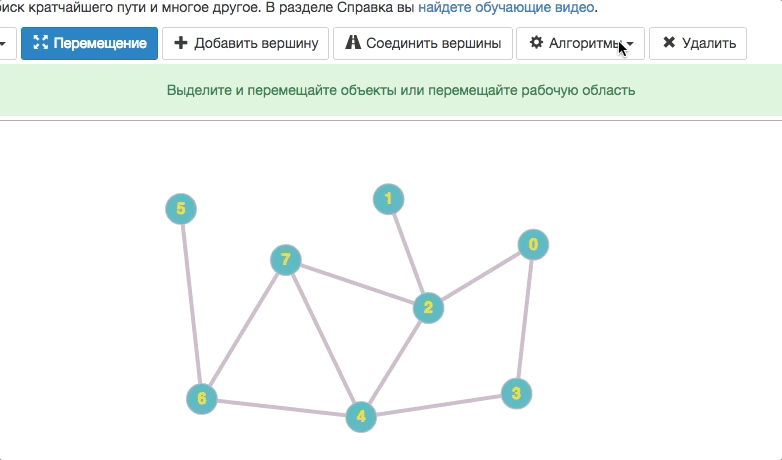Help

# Searching for graph’s radius and diameter

## Specifications

The eccentricity of a graph vertex is the maximum distance till the distant vertex.

The radius of a graph is the minimum eccentricity among all the vertexes of a graph.

The diameter of a graph is the longest distance among all the pairs of the graph’s vertexes.

The central vertex of a graph is the vertex which eccentricity equals the graph’s radius.

The peripheral vertex of a graph is the vertex which eccentricity equals the graph’s diameter.

## Searching for radius and diameter

Graph Online allows users to find radius and diameter. Moreover, it will point out the central and peripheral vertexes. In order to do it choose “Algorithms” and then “Search graph radius and diameter”.Implementation of the algorithm for JavaScript could be found at http://graphonline.ru/script/plugins/RadiusAndDiameter.js